### 2.5 Eigen­value Prob­lems

To an­a­lyze quan­tum me­chan­i­cal sys­tems, it is nor­mally nec­es­sary to find so-called eigen­val­ues and eigen­vec­tors or eigen­func­tions. This sec­tion de­fines what they are.

A nonzero vec­tor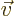is called an eigen­vec­tor of a ma­trix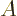if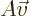is a mul­ti­ple of the same vec­tor: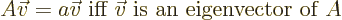(2.13)

The mul­ti­ple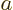is called the eigen­value. It is just a num­ber.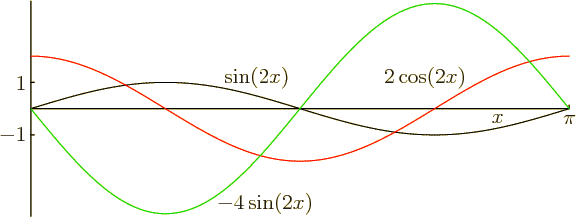A nonzero func­tionis called an eigen­func­tion of an op­er­a­torif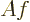is a mul­ti­ple of the same func­tion: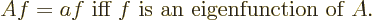(2.14)

For ex­am­ple,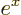is an eigen­func­tion of the op­er­a­tor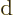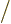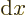with eigen­value 1, since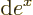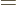1. An­other sim­ple ex­am­ple is il­lus­trated in fig­ure 2.8; the func­tion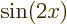is not an eigen­func­tion of the first de­riv­a­tive op­er­a­tor. How­ever it is an eigen­func­tion of the sec­ond de­riv­a­tive op­er­a­tor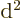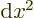, and with eigen­value4.

Eigen­func­tions likeare not very com­mon in quan­tum me­chan­ics since they be­come very large at large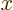, and that typ­i­cally does not de­scribe phys­i­cal sit­u­a­tions. The eigen­func­tions of the first de­riv­a­tive op­er­a­torthat do ap­pear a lot are of the form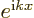, where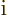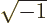and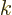is an ar­bi­trary real num­ber. The eigen­value is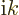: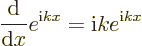Func­tiondoes not blow up at large; in par­tic­u­lar, the Euler for­mula (2.5) says: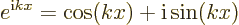The con­stantis called the “wave num­ber.”

Key Points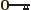If a ma­trix turns a nonzero vec­tor into a mul­ti­ple of that vec­tor, then that vec­tor is an eigen­vec­tor of the ma­trix, and the mul­ti­ple is the eigen­value.If an op­er­a­tor turns a nonzero func­tion into a mul­ti­ple of that func­tion, then that func­tion is an eigen­func­tion of the op­er­a­tor, and the mul­ti­ple is the eigen­value.

2.5 Re­view Ques­tions
1.

Show that, above, is also an eigen­func­tion of, but with eigen­value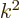. In fact, it is easy to see that the square of any op­er­a­tor has the same eigen­func­tions, but with the square eigen­val­ues.

2.

Show that any func­tion of the form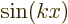and any func­tion of the form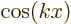, whereis a con­stant called the wave num­ber, is an eigen­func­tion of the op­er­a­tor, though they are not eigen­func­tions of.

3.

Show thatand, witha con­stant, are eigen­func­tions of the in­ver­sion op­er­a­tor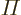, which turns any func­tion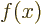into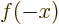, and find the eigen­val­ues.